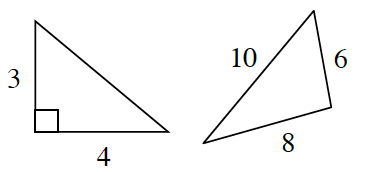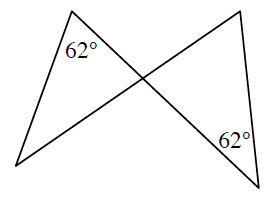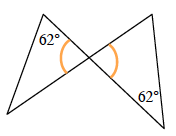### Home > CCG > Chapter 6 > Lesson 6.1.4 > Problem6-35

6-35.

For each pair of triangles below, decide if the pair is similar, congruent or neither. Justify your conclusion using a flowchart. Assume that the diagrams are not drawn to scale. Redraw and label the diagrams as needed.

Note: In this course, you are expected to label the vertices of your triangles.

1.Find the length of the hypotenuse of the right triangle.

1.What kind of angles are these?Since the vertical angles are congruent, the triangles are similar by $\text{AA}\sim$.